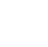Back to Chapter
Java IntroductionJava BasicsBranching and LoopingClasses, Objects, and MethodsArrays, Strings, and VectorsPackagesErrors and ExceptionApplet ProgrammingGraphics ProgrammingManaging I/O FilesJava Database Connectivity# Java Arrays, Strings, and Vectors Exercise

## Problem Statement-1:

Write a Java program to find the number of even and odd integers in a given array of integers.

Test Data:

array_nums = {5, 7, 2, 4, 9}

Expected output:

Original Array: [5, 7, 2, 4, 9]Number of even numbers: 2Number of odd numbers: 3

Solution:

import java.util.Arrays;
public class ArrayExample {
public static void main(String[] args) {
int[] array_nums = {
5,
7,
2,
4,
9
};
System.out.println("Original Array: " + Arrays.toString(array_nums));
int num = 0;
for (int i = 0; i < array_nums.length; i++) {
if (array_nums[i] % 2 == 0)
num++;
}
System.out.println("Number of even numbers : " + num);
System.out.println("Number of odd numbers  : " + (array_nums.length - num));
}
}

Solution:

Original Array: [5, 7, 2, 4, 9]
Number of even numbers: 2
Number of odd numbers: 3

Explanation:

The class ArrayExample consists of  an array int[] array_nums = { 5,7,2,4,9};. Using for loop the elements of the array are iterated. When the condition if (array_nums[i] % 2 == 0) is true the loop iterates,then the variable num holds the count of even numbers and array_nums.length - num holds the count of odd numbers.

## Problem Statement-2:

Write a Java program to convert all the characters in a string to lowercase.

Test Data:

str = "The Quick Brown Fox!"

Expected output:

Original String: The Quick Brown Fox!
String in lowercase: the quick brown fox!

Solution:

public class StringExercise {
public static void main(String[] args)
{
String str = "The Quick Brown Fox!";

// Convert the above string to all lowercase.
String lowerStr = str.toLowerCase();

// Display the two strings for comparison.
System.out.println("Original String: " + str);
System.out.println("String in lowercase: " + lowerStr);
}
}

Output:

Original String: The Quick Brown Fox!
String in lowercase: the quick brown fox!

Explanation:

The class StringExercise contains the string variable str. The string variable is assigned as

str = "The Quick Brown Fox!".The method toLowerCase() is used to convert the string into lowercase. The println() method is used to print the statement.